# Eddy currents in a conductive disc

HOME / Applications / Eddy currents in a conductive disc

### Applications

Used Tools:## Physics

A cylindrical region contains homogenous, time varying magnetic field; B0 is the field magnitude andis vertical unit vector. Since there is no electric charge in the system, the changing magnetic field is the sole cause of the electric field. According to Farady's equation :(eq.1)

or in the case whereonly has z component:(eq.2)

where  r andrepresent radial and angular coordinates of the cylindrical coordinate system (Fig. 1).

Due to the symmetry of the problem, its analysis can be simplified by noting thatdoes not depend on, i.e.. Equation 2 therefore, takes the following form:(eq.3)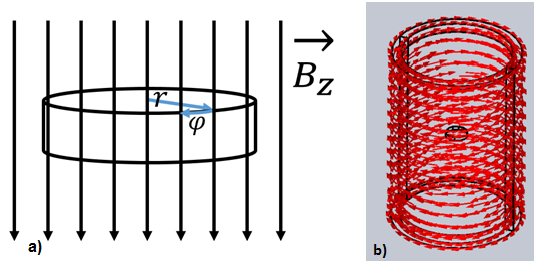Figure 1 - a) Copper disc in vertical magnetic field;b) Changing magnetic field is produced inside a cylinder wrapped in coils that carry AC current;

To solve for, equation 3 is multiplied byand then integrated from 0 toas:orIf a copper disc is placed inside the cylinder, as a consequence of induced electric field, eddy currents are distributed inside the disc. Current densityis, according to the Ohm’s law :whereis specific conductivity of copper (). For a magnetic field with a magnitude ofand angular frequency, magnitude of  current density is. Induced eddy currents lag the change in flux density by 90º.

## Model

Eddy current distribution in a copper disc can be easily simulated in EMS as a AC Magnetic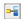study. To create uniform magnetic field inside cylinder, allow certain thickness to its wall, so that you can define the wall as a wound coil.Cylinder with inner radius of 15mm and height of 50mm, should be used to define Wound Coil with 100 turns and RMS current magnitude per turn of.For Current phase select 0º - this will produce cosine current profile in the coil. This current will in turn induce relatively uniform flux density of magnitudeover the volume of the copper disc (radius: 3mm), placed in the center of the cylinder. To help magnetic field align vertically inside the cylinder, add Normal Flux boundary condition to cylinder caps and Tangential Flux  boundary condition to the inner face of the cylinder wall.

## Boundary conditions

To help magnetic field align vertically inside the cylinder, Normal Flux boundary condition to cylinder caps and Tangential Flux boundary condition to the inner face of the cylinder wall, should be added.

To do define the Normal Flux,
1. In the EMS manger tree right-click on the Load/Restraint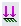folder and select Normal Flux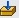.
2. Click inside the Faces for Normal Flux box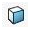then select Cylinder caps .
3. Click OK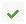.
To do define the Tangential Flux,
1. In the EMS manger tree right-click on the Load/Restraintfolder and select Tangential Flux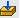.
2. Click inside the Faces for Tangential Fluxbox  then select the inner face of the Cylinder wall .
3. Click OK.

### Coils

To show how to define the Wound Coil, see “Force in a magnetic circuit” example.

### Eddy Effects

To set up Eddy Effects, right click the copper disc in the EMS tree and select Turn on Eddy Effects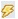.

### Meshing

To get high resolution of current density results in the copper disc, a Mesh control of 0.1 mm should be applied on the copper disc.
To do so,

1. In the EMS manger tree right-click on the Mesh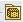folder and select Apply Mesh Control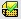.
2. Click inside the Bodies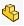box then select the inner face of the copper disc .
3. Under Control Parameters click inside the Element Size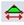box and type 0.1 mm.
4. Click OK.

To mesh the model:

1. In the EMS manger tree, right-click on the Mesh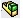icon and select Create Mesh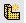.
2. Click OK.

## Results

To plot current density distribution in the copper disc, right click the Current Density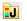in the EMS tree and select 2D Plot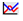. For the current density Representation select Real (instantaneous). Maximum current density occurs for 90º  (minimum at 270º ), since the eddy currents have sinusoidal time dependence (in case of a cosine coil current and magnetic field).It is enough to select only 2 points along the disc radius – one in the center and the other one by the periphery. Type in number of points you want in between and EMS will plot current density along the disc radius.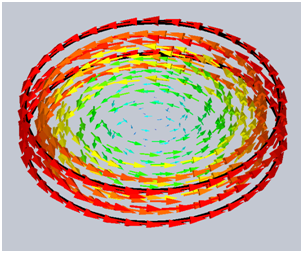Figure 2 - 3D vector plot of Eddy current density inside the copper disc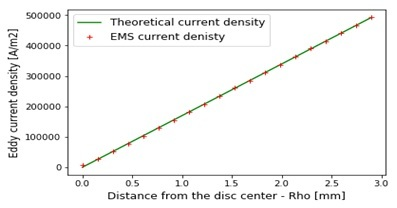Figure 3 - Comparison of EMS and theoretical results for eddy current density

The agreement between the theoretical solution () and the EMS 2D current density plot is displayed on Figure 3.